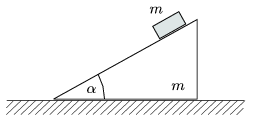Mathematical and Physical Journal
for High Schools
Issued by the MATFUND Foundation
 Already signed up? New to KöMaL?

#Problem P. 4097. (October 2008)

P. 4097. The wedge, shown in the figure, has a mass of m and an angle ofand can slide without friction along the horizontal tabletop. A block of mass m is placed onto the wedge, and it slides down without friction. At what anglewill the magnitude of the acceleration of the wedge be at least one-third of the acceleration due to gravity?(5 pont)

Deadline expired on November 11, 2008.

### Statistics:

 158 students sent a solution. 5 points: 54 students. 4 points: 9 students. 3 points: 34 students. 2 points: 37 students. 1 point: 9 students. 0 point: 15 students.

Problems in Physics of KöMaL, October 2008